1 Starting Out: Elementary Arithmetic
 In:=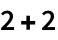Out=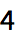In:=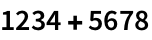Out=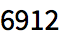In:=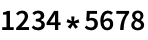Out=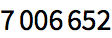1.1Compute 1+2+3. »
Expected output:
 Out[]=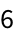Answer & check your solution
1.2Add the numbers 1, 2, 3, 4, 5. »
Expected output:
 Out[]=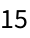Answer & check your solution
1.3Multiply the numbers 1, 2, 3, 4, 5. »
Expected output:
 Out[]=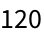Answer & check your solution
1.4Compute 5 squared (i.e. 5×5 or 5 raised to the power 2). »
Expected output:
 Out[]=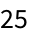Answer & check your solution
1.5Compute 3 raised to the fourth power. »
Expected output:
 Out[]=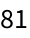Answer & check your solution
1.6Compute 10 raised to the power 12 (a trillion). »
Expected output:
 Out[]=Answer & check your solution
1.7Compute 3 raised to the power 7×8. »
Expected output:
 Out[]=Answer & check your solution
1.8Add parentheses to 4-2*3+4 to make 14. »
Expected output:
 Out[]=Answer & check your solution
Expected output:
 Out[]=Answer & check your solution
+1.1Add all integers from -3 to +3.  »
Expected output:
 Out[]=Answer & check your solution
+1.2Compute 24 divided by 3. »
Expected output:
 Out[]=Answer & check your solution
+1.3Compute 5 raised to the power 100. »
Expected output:
 Out[]=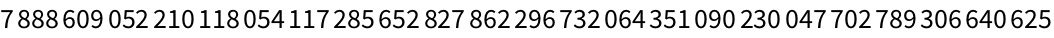Answer & check your solution
+1.4Subtract 5 squared from 100. »
Expected output:
 Out[]=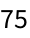Answer & check your solution
+1.5Multiply 6 by 5 squared, and add 7. »
Expected output:
 Out[]=Answer & check your solution
+1.6Compute 3 squared minus 2 cubed.  »
Expected output:
 Out[]=Answer & check your solution
+1.7Compute 2 cubed times 3 squared. »
Expected output:
 Out[]=Answer & check your solution
+1.8Compute double the sum of eight and negative eleven»
Expected output:
 Out[]=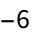Answer & check your solution
In 2+2, etc. how do I tell the Wolfram Language that Ive finished my input?
Why is multiplication indicated by *?
6^3 means 6×6×6 (i.e. 6 multiplied by itself 3 times); 10^5 means 10×10×10×10×10; etc.
How big can numbers get in the Wolfram Language?
The same as in ordinary math: powers, multiplication, addition. So 4*5^2+7 means (4*(5^2))+7. You can use parentheses just like in math. (In math, people sometimes use [...] as well as (...). In the Wolfram Language [...] means something different.)
When I do division, how do I avoid getting fractions in my answer?
What are the little spaces between digits in results like 7006652?
Theyre there to help you read the number when its displayed; theyre not part of the number.
How do I enter a big number?
Just type the digits, without putting any commas, spaces or other separators (e.g. 1234123511415223).
What happens if I compute 1/0?